## 4.2.3 Chains and Trees of Bodies

If there are multiple bodies that are allowed to move independently, then their C-spaces can be combined using Cartesian products. Let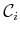denote the C-space of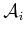. If there arefree-floating bodies in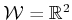or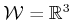, then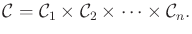(4.32)

If the bodies are attached to form a kinematic chain or kinematic tree, then each C-space must be considered on a case-by-case basis. There is no general rule that simplifies the process. One thing to generally be careful about is that the full range of motion might not be possible for typical joints. For example, a revolute joint might not be able to swing all of the way around to enable any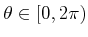. If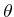cannot wind around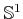, then the C-space for this joint is homeomorphic toinstead of. A similar situation occurs for a spherical joint. A typical ball joint cannot achieve any orientation in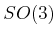due to mechanical obstructions. In this case, the C-space is not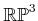because part ofis missing.

Another complication is that the DH parameterization of Section 3.3.2 is designed to facilitate the assignment of coordinate frames and computation of transformations, but it neglects considerations of topology. For example, a common approach to representing a spherical robot wrist is to make three zero-length links that each behave as a revolute joint. If the range of motion is limited, this might not cause problems, but in general the problems would be similar to using yaw, pitch, and roll to represent. There may be multiple ways to express the same arm configuration.

Several examples are given below to help in determining C-spaces for chains and trees of bodies. Suppose, and there is a chain ofbodies that are attached by revolute joints. Suppose that the first joint is capable of rotation only about a fixed point (e.g., it spins around a nail). If each joint has the full range of motion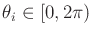, the C-space is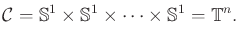(4.33)

However, if each joint is restricted to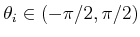, then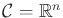. If any transformation in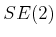can be applied to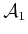, then an additional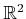is needed. In the case of restricted joint motions, this yields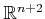. If the joints can achieve any orientation, then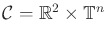. If there are prismatic joints, then each joint contributesto the C-space.

Recall from Figure 3.12 that forthere are six different kinds of joints. The cases of revolute and prismatic joints behave the same as for. Each screw joint contributes. A cylindrical joint contributes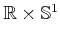, unless its rotational motion is restricted. A planar joint contributes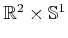because any transformation inis possible. If its rotational motions are restricted, then it contributes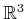. Finally, a spherical joint can theoretically contribute. In practice, however, this rarely occurs. It is more likely to contributeorafter restrictions are imposed. Note that if the first joint is a free-floating body, then it contributes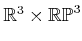.

Kinematic trees can be handled in the same way as kinematic chains. One issue that has not been mentioned is that there might be collisions between the links. This has been ignored up to this point, but obviously this imposes very complicated restrictions. The concepts from Section 4.3 can be applied to handle this case and the placement of additional obstacles in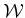. Reasoning about these kinds of restrictions and the path connectivity of the resulting space is indeed the main point of motion planning.

Steven M LaValle 2020-08-14